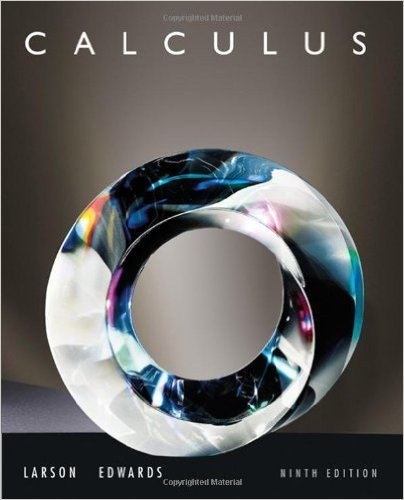×
×

# Solutions for Chapter 10.3: Parametric Equations and Calculus## Full solutions for Calculus | 9th Edition

ISBN: 9780547167022Solutions for Chapter 10.3: Parametric Equations and Calculus

Solutions for Chapter 10.3
4 5 0 237 Reviews
29
5
##### ISBN: 9780547167022

Chapter 10.3: Parametric Equations and Calculus includes 105 full step-by-step solutions. Calculus was written by and is associated to the ISBN: 9780547167022. This expansive textbook survival guide covers the following chapters and their solutions. This textbook survival guide was created for the textbook: Calculus , edition: 9. Since 105 problems in chapter 10.3: Parametric Equations and Calculus have been answered, more than 61658 students have viewed full step-by-step solutions from this chapter.

Key Calculus Terms and definitions covered in this textbook
• Absolute minimum

A value ƒ(c) is an absolute minimum value of ƒ if ƒ(c) ? ƒ(x)for all x in the domain of ƒ.

• Annual percentage rate (APR)

The annual interest rate

• Elementary row operations

The following three row operations: Multiply all elements of a row by a nonzero constant; interchange two rows; and add a multiple of one row to another row

• First octant

The points (x, y, z) in space with x > 0 y > 0, and z > 0.

• Horizontal asymptote

The line is a horizontal asymptote of the graph of a function ƒ if lim x:- q ƒ(x) = or lim x: q ƒ(x) = b

• Instantaneous rate of change

See Derivative at x = a.

• Length of a vector

See Magnitude of a vector.

• Linear regression equation

Equation of a linear regression line

• Linear system

A system of linear equations

• Median (of a data set)

The middle number (or the mean of the two middle numbers) if the data are listed in order.

• Nautical mile

Length of 1 minute of arc along the Earth’s equator.

• Polar coordinates

The numbers (r, ?) that determine a point’s location in a polar coordinate system. The number r is the directed distance and ? is the directed angle

An equation that can be written in the form ax 2 + bx + c = 01a ? 02

• Quartic function

A degree 4 polynomial function.

• Remainder theorem

If a polynomial f(x) is divided by x - c , the remainder is ƒ(c)

• Sample space

Set of all possible outcomes of an experiment.

• Solution of a system in two variables

An ordered pair of real numbers that satisfies all of the equations or inequalities in the system

• Third quartile

See Quartile.

• Translation

See Horizontal translation, Vertical translation.

• Vertex of a parabola

The point of intersection of a parabola and its line of symmetry.

×